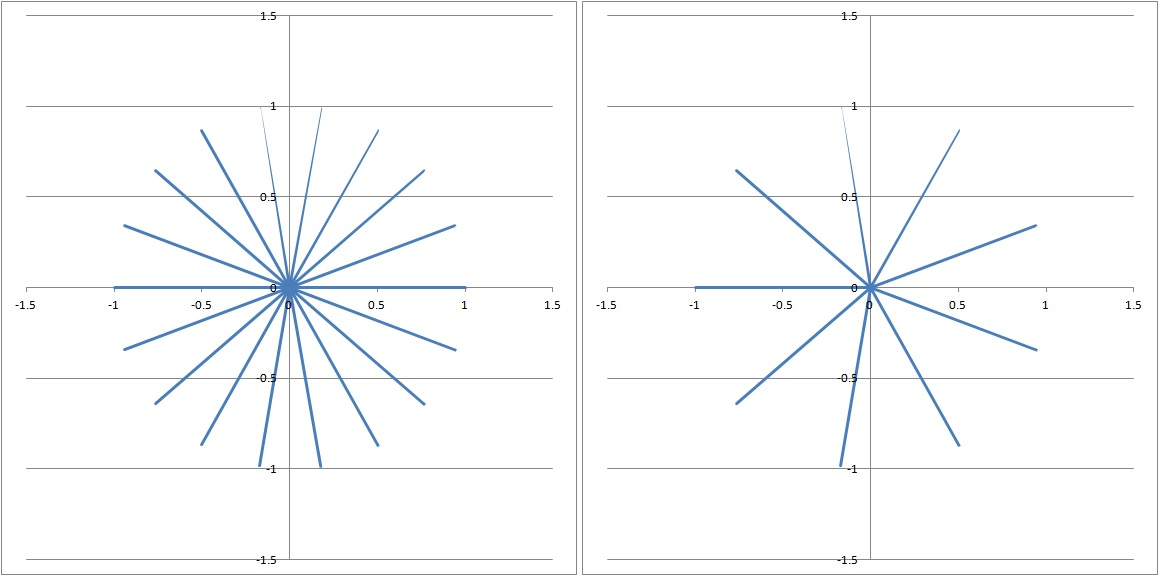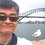# Argand Diagram and $\sum_{k-0}^{n-1} \cos \left(\frac {2k+1}{2n+1}\pi \right) = \frac 12$In a few of my solutions for trigonometry problems, I mentioned that Argand diagram shows that:

• $\displaystyle \sum_{k=1}^{\lfloor \frac{n}{2} \rfloor} \cos{\frac{(2k-1)\pi}{n}} = \frac{1}{2}$ and
• $\displaystyle \sum_{k=1}^{\lfloor \frac{n}{2} \rfloor} \cos{\frac{2k\pi}{n}} = -\frac{1}{2}$,

where $n$ is an odd integer, without clearly explaining it. This note explains it.

Without loss of generality, let us use $n=9$. Then we note that $\cos{\frac{k\pi}{9}}$, where $k = 0,2,3,...17$ are the real part of $\omega^{18}$ the $18^{th}$ roots of unity, as $e^{\frac{k\pi}{9}} = \cos{\frac{k\pi}{9}} + i \sin{\frac{k\pi}{9}}$.

Therefore, they can be represented by a Argand diagram (left). It is easy to see from the Argand diagram that:

• $\displaystyle \sum_ {k=0}^{17} \cos{\frac{k\pi}{9}} = 0$ and
• $\displaystyle \sum_ {k=0}^{17} \sin{\frac{k\pi}{9}} = 0$

because for every root $\omega^i$ there is an opposite $-\omega^{9+i}$.

The Argand diagram shows the fact that $\displaystyle \sum_ {k=0}^{n-1} \omega^k = 0$, which is true for $\omega^{n} = 1$.

Now, if we remove the even roots $k = 0, 2, 4, ... 16$. The Argand diagram (right) is left with 9 uniform distributed odd roots. Does the Argand diagram shows that:

$\displaystyle \sum_{k=1}^{\color{#D61F06}{9}} \omega^{2k-1} = 0$?

Yes, it does. We can check it out numerically. But can we explain it without using numerical method? Yes, I have a way which does not resort to serious algebra but classical mechanics.

Let the real and imaginary axes be replaced by $x$- and $y$ axes on a horizontal plane respectively. Let the 9 roots be identical thin solid rod of unit length each with mass $m$ and are connected firmly at the center, like the spokes of a wheel without a rim. Let the wheel be pivoted freely at the center. We can expect it to be balanced and stay horizontal because the rods are symmetrically distributed from the center. This means that the resultant moment of the wheel on any horizontal axis through the center is $0$. If we take the moments about $y$-axis, then the resultant moment:

$\displaystyle \sum_{k=1}^{9} \frac{1}{2}\cos{\frac{(2k-1)\pi}{9}}mg = 0$,

where $g$ is acceleration due to gravity and the factor $\frac{1}{2}$ is because center of mass is at the middle of the rod.

This implies that :

• $\displaystyle \sum_{k=1}^{9} \cos{\frac{(2k-1)\pi}{9}} = 0$ and similarly,
• $\displaystyle \sum_{k=1}^{9} \sin{\frac{(2k-1)\pi}{9}} = 0$ and therefore,
• $\displaystyle \sum_{k=1}^{9} \omega^{2k-1} = 0$.

We can conclude that if the complex numbers represented by an Argand diagram is symmetrical from the center the sum of the complex number is $0$.

We know that $\cos{\frac{k\pi}{9}} = \cos{\frac{-k\pi}{9}} = \cos{\frac{(18-k)\pi}{9}}$, therefore,

\begin{aligned} \sum_{k=1}^{9} \cos{\frac{(2k-1)\pi}{9}} & = 0 \\ 2 \sum_{k=1}^{4} \cos{\frac{(2k-1)\pi}{9}} - \cos{\pi }& = 0 \\ 2 \sum_{k=1}^{4} \cos{\frac{(2k-1)\pi}{9}} - 1 & = 0 \\ \Rightarrow \sum_{k=1}^{4} \cos{\frac{(2k-1)\pi}{9}} & = \frac{1}{2} \end{aligned}

Therefore, showing $\displaystyle \sum_{k=1}^{\lfloor \frac{n}{2} \rfloor} \cos{\frac{(2k-1)\pi}{n}} = \frac{1}{2}$, when $n$ is an odd integer. Similar explanation can be used for $\displaystyle \sum_{k=1}^{\lfloor \frac{n}{2} \rfloor} \cos{\frac{2k\pi}{n}} = -\frac{1}{2}$.Note by Chew-Seong Cheong
5 years, 11 months ago

This discussion board is a place to discuss our Daily Challenges and the math and science related to those challenges. Explanations are more than just a solution — they should explain the steps and thinking strategies that you used to obtain the solution. Comments should further the discussion of math and science.

When posting on Brilliant:

• Use the emojis to react to an explanation, whether you're congratulating a job well done , or just really confused .
• Ask specific questions about the challenge or the steps in somebody's explanation. Well-posed questions can add a lot to the discussion, but posting "I don't understand!" doesn't help anyone.
• Try to contribute something new to the discussion, whether it is an extension, generalization or other idea related to the challenge.
• Stay on topic — we're all here to learn more about math and science, not to hear about your favorite get-rich-quick scheme or current world events.

MarkdownAppears as
*italics* or _italics_ italics
**bold** or __bold__ bold
- bulleted- list
• bulleted
• list
1. numbered2. list
1. numbered
2. list
Note: you must add a full line of space before and after lists for them to show up correctly
paragraph 1paragraph 2

paragraph 1

paragraph 2

[example link](https://brilliant.org)example link
> This is a quote
This is a quote
    # I indented these lines
# 4 spaces, and now they show
# up as a code block.

print "hello world"
# I indented these lines
# 4 spaces, and now they show
# up as a code block.

print "hello world"
MathAppears as
Remember to wrap math in $$ ... $$ or $ ... $ to ensure proper formatting.
2 \times 3 $2 \times 3$
2^{34} $2^{34}$
a_{i-1} $a_{i-1}$
\frac{2}{3} $\frac{2}{3}$
\sqrt{2} $\sqrt{2}$
\sum_{i=1}^3 $\sum_{i=1}^3$
\sin \theta $\sin \theta$
\boxed{123} $\boxed{123}$

## Comments

Sort by:

Top Newest

(√2+i√2/3)^(1+i),what is the value of it ?

- 5 years, 10 months ago

Log in to reply

\begin{aligned} \left( \sqrt{2} + i\frac{\sqrt{2}}{3} \right)^{1+i} & = \left(\frac{\sqrt{20}}{3} \right)^{1+i} \left( \frac{3}{\sqrt{10}} + i\frac{1}{\sqrt{10}} \right)^{1+i} = \left(\frac{\sqrt{20}}{3} \right) \left( e^{i\ln{\frac{\sqrt{20}}{3}}} \right) \left( e^{i \tan^{-1}\frac{1}{3}} \right)^{1+i} \\ & = \frac {\sqrt{20} e^{i\ln{\frac{\sqrt{20}}{3}}} e^{\tan^{-1}\frac{1}{3}(-1+i)}} {3} = \frac {\sqrt{20} e^{i\ln{\frac{\sqrt{20}}{3}}} e^{i\tan^{-1}\frac{1}{3}}} {3e^{\tan^{-1}\frac{1}{3}}} \\ & = \frac {\sqrt{20}e^{i\ln{\frac{\sqrt{20}}{3}}}} {3e^{\tan^{-1}\frac{1}{3}}} \left( \frac{3}{\sqrt{10}} + i\frac{1}{\sqrt{10}} \right) \\ & = \frac {\sqrt{2}\left(\cos{\left(\ln{\frac{\sqrt{20}}{3}}\right)} +i \sin{\left(\ln{\frac{\sqrt{20}}{3}}\right)}\right)(3+i)} {3e^{\tan^{-1}\frac{1}{3}}} \\ & = \boxed{0.811674521 + i0.713337315} \end{aligned}

- 5 years, 10 months ago

Log in to reply

amazing.great piece of information! :))

- 5 years ago

Log in to reply

×

Problem Loading...

Note Loading...

Set Loading...